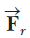# Problem: The potential energy of a system of two particles separated by a distance r is given by U(r) = A/r, where A is a constant. Find the radial force  that each particle exerts on the other.

⚠️Our tutors found the solution shown to be helpful for the problem you're searching for. We don't have the exact solution yet.

###### Problem Details

The potential energy of a system of two particles separated by a distance r is given by U(r) = A/r, where is a constant. Find the radial forcethat each particle exerts on the other.